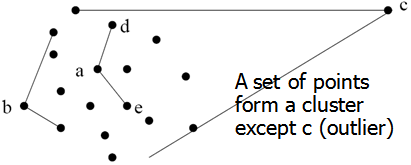# Current Slide

Small screen detected. You are viewing the mobile version of SlideWiki. If you wish to edit slides you will need to use a larger device.

### Approach III: Modeling High-Dimensional Outliers

• Ex. Angle-based outliers: Kriegel, Schubert, and Zimek [KSZ08]
• For each point o, examine the angle ∆xoy for every pair of points x, y.
• Point in the center (e.g., a), the angles formed differ widely
• An outlier (e.g., c), angle variable is substantially smaller• Use the variance of angles for a point to determine outlier
• Combine angles and distance to model outliers
• Use the distance-weighted angle variance as the outlier score
• Angle-based outlier factor (ABOF):

$ABOF(o)=VAR_{x,y\epsilon D,x\neq o,y\neq o}\frac{<\vec{ox},\vec{oy} > }{dist(o,x)^2dist(o,y)^2}$

• Efficient approximation computation method is developed
• It can be generalized to handle arbitrary types of data
• Develop new models for high-dimensional outliers directly
• Avoid proximity measures and adopt new heuristics that do not deteriorate in high-dimensional data

Speaker notes:

## Content Tools

### Sources

There are currently no sources for this slide.